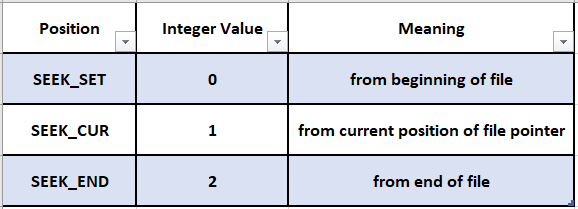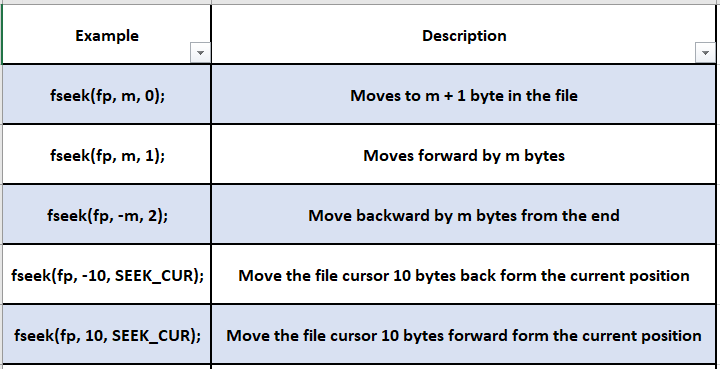In this tutorial, we are going to learn about C file operations.

## C File Operations:

The functions getc() and fgetc() are used to read a character from the file which is opened for reading purpose. Both the functions have the same syntax and their work is also similar.

## Read and Write characters using getc() and putc():

The general syntax of getc() is:

``int getc(FILE *fp);``

The getc() function returns the ASCII equivalent of the character (which is an integer) that is being read. If the getc() function detects it has reached the end of the file, it will return the special value EOF. If an `error` occurs while reading the file, the getc() function will set the error indicator and return EOF.
Consider the following example:

``````FILE *fp;
fp = fopen("sample.txt", "r");
ch= getc(fp);``````

Here, a single character is read from the file identified by the file pointer fp. That character is then assigned to character variable ch.
The functions putc() and fputc() are used to write a character into a file that is opened for writing.
The general syntax of putc() is:

``int putc(int c, FILE *fp);``

If an error occurs, it returns EOF.

Consider the following example:

``putc(ch, fp);``

Here, the statement will write the character specified by ch into the file pointed by fp.

## Read and Write integers using getw() and putw():

The function putw() is used to write an integer value to the file pointed by the file pointer.
The syntax of putw() is:

``putw(int number, FILE *fp);``

The putw() function takes two arguments, first is an integer value to be written to the file and the second is the file pointer where the number will be written.

The function getw() returns the integer value from a file and it increments the value of the address stored in the file pointer.

The syntax of getw() is:

``int getw(FILE *fp);``

The getw() function takes the file pointer as an argument from which the integer value is read and it returns EOF if it has reached the end of the file.

## Read and Write strings using fgets() and fputs():

C language supports functions for reading and writing strings from/to disk files respectively. The functions fgets() and fputs() are used for this purpose.

fputs() is used to write a string onto the file pointed by the file pointer.

The syntax of fputs() is as follows:

``char* fputs(char* str, FILE* fp);``

fputs() writes a string specified by str into the file pointed by fp.

fgets() is used to read a string from the file pointed by the file pointer.

The syntax of fgets() is as follows:

``char* fgets(char* str, int length, FILE* fp);``

fgets() reads the length in number of bytes from the file pointed by fp into the character array str.

Both the functions will return NULL upon unsuccessful execution.

## Read and Write mixed data values using fscanf() and fprintf():

C language provides two functions fprintf() and fscanf() for handling a set of mixed data values.

The function fprintf() is used to write a mix of different data items into a specified file.

Similarly, the function fscanf() is used to read a mix of different data items from a specified file.

The general syntax of fprintf() is:

``fprintf (FILE* fp, char* control_string, variables);``

Here fp is a file pointer associated with a file that has been opened for writingcontrol_string is the type specifier of the variables to be sent to the file pointed by fp.

The general syntax of fscanf() is as follows:

``fscanf (FILE* fp, char* control_string, address_of_variables);``

## Random File Accessing:

### 1. Using ftell() function:

If we want to access a particular record, C supports some functions for random access file processing.

In random access files, the data can be read and modified randomly. If the user interested in accessing only a part of a file then they can use these random access files.

C provides three functions to access data randomly. They are:

• ftell()
• fseek()
• rewind()

ftell() function determines the location of file pointer in the file.

The syntax of ftell() is as follows:

``long int ftell(FILE* fp);``

The ftell() function returns the byte number at which the file cursor is presently pointing to the file referenced by fp. The value is count from the beginning of the file.

Let us consider an example:

``int lvalue = ftell(fp);``

This statement will store the byte number pointed by the file pointer fp in the file and assigns that value to the variable lvalue.

### 2. Using rewind() function:

The rewind() function resets the file pointer to the starting position of the file.

The syntax of rewind is as follows:

``void rewind(FILE* fp);``

Consider the following example:

``rewind(fp);``

This statement will move the file cursor to the beginning of the file pointed by fp.

Thus rewind() can be used to go back to the starting of the file in any state of the program, by sending the file pointer as the parameter.

### 3. Using fseek() function:

The fseek() function is used to move the file position to the desired location within a file.

The syntax of fseek() function is as follows:

``int fseek(FILE* fp, long offset, int position);``

While fp is a file pointer, offset specifies the number of bytes (positions) to be moved from the location specified by position and position can be any of the following:The offset is positive for forwarding movement in the file and negative for backward movement.

Consider the following examples to understand the usage of fseek():When an operation is successful the fseek() returns a zero. If the user attempts to move the file pointer beyond the file boundaries an error occurs and fseek() returns -1.

Happy Learning 🙂

### About the Author: Hemanth Krishna Vineel RamayanamI’m an Undergraduate Student at the Indian Institute of Information Technology, Design, and Manufacturing, Kurnool, Andhra Pradesh. I belong to the CSE domain.